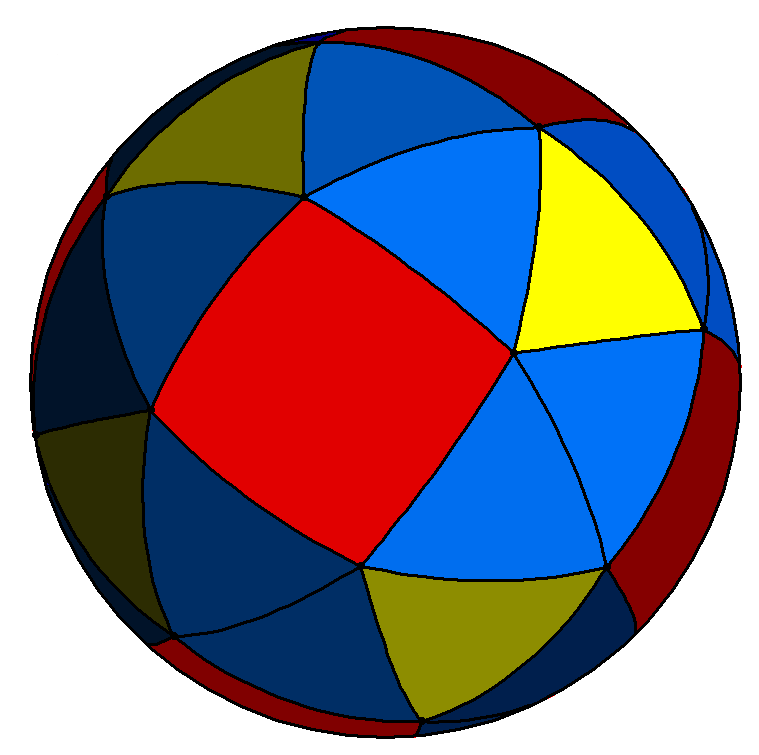# Square on a Sphere

Geometry Level 5A square is drawn on the surface of a sphere. The radius of the sphere is 100. If each interior angle of the square measures 100 degrees, what is the area of the spherical square?

×